# How to write a union symbol(∪) in LaTeX?

An important concept in set operation is union operation. Union symbols are used for this union operation. And this union symbol is marked by ∪.

Properties Value
Symbol Union
Argument No
Command \cup
Example \cup → ∪

With the help of latex, you need to use the \cup command to represent union symbols in a scientific document. So, look at this example below

\documentclass{article}
\begin{document}
$$A \cup B = \{x : x\in A \; or \; x\in B \}$$
$$A \cup \phi \; or \; A\cup \{\} = A$$
$$(A_1 \cup A_2 \cup A_3 \cup \cdots A_n)^c=A_1^c \cap A_2^c \cap A_3^c\cdots \cap A_n^c$$
$$A\cup (B\cup C)=(A\cup B)\cup C$$
\end{document}

Output :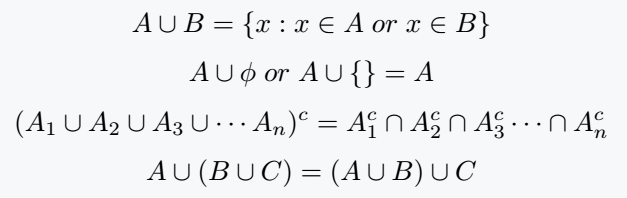## Big union symbol in LaTeX

When a union is defined between more than three sets, it is represented in the form of n summation. However, the only difference is that the union symbol is used instead of the summation symbol.

\documentclass{article}
\begin{document}
$$\cup_{i=1}^{n}F_{i}$$
\end{document}

Output :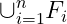If you look at the output above, you will see that the lower limit and the upper limit are not located above and below the union symbol. Thus, the above latex union symbol is not the best practice for union operations of n number sets.

For this you need to use the Big Union symbol. And you have to use the limits above and below this Big Union symbol. So, look at this program below

\documentclass{article}
\begin{document}
$$\bigcup_{i=1}^{n}F_{i}$$
\end{document}

Output :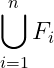In latex, you need to use the \bigcup command for the big union symbol. And there is no need to install any external package in latex for this command.

You can add upper and lower limits using the \limits command with Big Union.

\documentclass{article}
\begin{document}
$$\bigcup\limits_{i=1}^{n}F_{i}$$
\end{document}

Output :In the case of n number sets, in my opinion, the last two methods are the best practice.

## LaTeX disjoint union symbol

Generally, disjoint union symbols in latex are characterized by the following three methods.

1. You can represent a disjoint union symbol in a document by using a dot symbol over the union symbol. For this you need to use \dot\cup command.

\documentclass{article}
\begin{document}
$$S_{1} \dot\cup S_{2}$$
\end{document}

Output :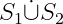2. Second, you can use the \uplus command. And this will add a plus symbol to the union symbol and return the output to you.

\documentclass{article}
\begin{document}
$$S_{1} \uplus S_{2}$$
\end{document}

Output :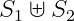3. Third, you can use four corner u shape symbols.

\documentclass{article}
\begin{document}
$$S_{1} \sqcup S_{2}$$
\end{document}

Output :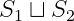You can use the disjoint union symbol document by installing an external latex package without using the above three default methods.#### Md Jidan Mondal

LaTeX expert with over 10 years of experience in document preparation and typesetting. Specializes in creating professional documents, reports, and presentations using LaTeX.